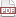### Intercoder agreement: the R script and its tests

12 July 14. [link]PDF version

Here, I will present an R script to calculate an information-based measure of intercoder agreement.

The short version: we have two people putting the same items into bins, and want to know how often they are in agreement about the bins. It should be complexity-adjusted, because with only two bins, binning at random achieves 50% agreement, while with 100 bins binning at random produces 1% agreement. We can use mutual information as a sensible measure of the complexity-adjusted agreement rate. A few more steps of logic, and we have this paper in the Journal of Official Statistics describing $P_i$, a measure of intercoder agreement via information in agreement. I also blogged this paper in a previous episode.

There are two features of the paper that are especially notable for our purposes here. The first is that I said that the code is available upon request. Somebody called me out on that, so I sent him the code below. Second, the paper has several examples, each with two raters and a list of their choices, and a carefully verified calculation of $P_i$. That means that the tests are already written.

The code below has two functions. We could turn it into a package, but it's not even worth it: just source("p_i.R") and you've got the two defined functions. The p_i function does the actual calculation, and test_p_i runs tests on it. As in the paper, some of the tests are extreme cases like full agreement or disagreement, and others are average tests that I verified several times over the course of writing the paper.

Could it be better? Sure: I don't do a very good job of testing the code for really pathological cases, like null inputs or something else that isn't a matrix. But the tests give me a lot of confidence that the p_i function does the correct thing given well-formed inputs. It's not mathematically impossible for a somehow incorrect function to give correct answers for all six tests, but with each additional test the odds diminish.

Here is the code. Feel free to paste it into your projects, or fork it from Github and improve upon it—I'll accept pull requests with improvements.


p_i <- function(dataset, col1=1, col2=2){

entropy <- function(inlist){
-sum(sapply(inlist, function(x){log2(x)*x}), na.rm=TRUE)
}

information_in_agreement <- function(diag, margin1, margin2){
sum <- 0
for (i in 1:length(diag))
if (diag[i] != 0)
sum <- sum + diag[i]*log2(diag[i]/(margin1[i]*margin2[i]))
return (sum)
}

dataset <- as.data.frame(dataset) #in case user provided a matrix.
crosstab <- table(as.data.frame(cbind(dataset[,col1],dataset[,col2])))
d1tab <- table(dataset[,col1])
d2tab <- table(dataset[,col2])
d1tab <- d1tab/sum(d1tab)
d2tab <- d2tab/sum(d2tab)
crosstab <- crosstab/sum(crosstab)

entropy_1 <- entropy(d1tab)
entropy_2 <- entropy(d2tab)
ia <- information_in_agreement(diag(crosstab), d1tab, d2tab)
return (2*ia/(entropy_1+entropy_2))
}

test_p_i <- function(){
fullagreement <- matrix(
c(1,1,1,1,2,2,2,2,3,3,
1,1,1,1,2,2,2,2,3,3),
ncol=2, byrow=FALSE
)

stopifnot(p_i(fullagreement)==1)

noagreement <- matrix(
c(1,2,1,2,1,2,3,1,3,2,
2,1,3,1,2,3,2,2,1,3),
ncol=2, byrow=FALSE
)

stopifnot(p_i(noagreement)==0)

constant <- matrix(
c(1,1,1,1,1,1,
1,1,2,2,2,3),
ncol=2, byrow=FALSE
)

stopifnot(p_i(constant)==0)

neg_corr <- matrix(
c(1,1,1,1,1,2,2,2,2,2,
1,2,2,2,2,1,1,1,1,2),
ncol=2, byrow=FALSE
)

stopifnot(abs(p_i(neg_corr)- -.2643856) < 1e-6)

rare_agreement <- matrix(
c(1,1,1,2,1,2,2,2,3,3,
1,1,1,1,2,2,2,2,3,3),
ncol=2, byrow=FALSE
)

stopifnot(abs(p_i(rare_agreement)- .6626594) < 1e-6)

common_agreement <- matrix(
c(1,1,1,1,2,2,2,3,2,3,
1,1,1,1,2,2,2,2,3,3),
ncol=2, byrow=FALSE
)

stopifnot(abs(p_i(common_agreement)- 0.6130587) < 1e-6)
}